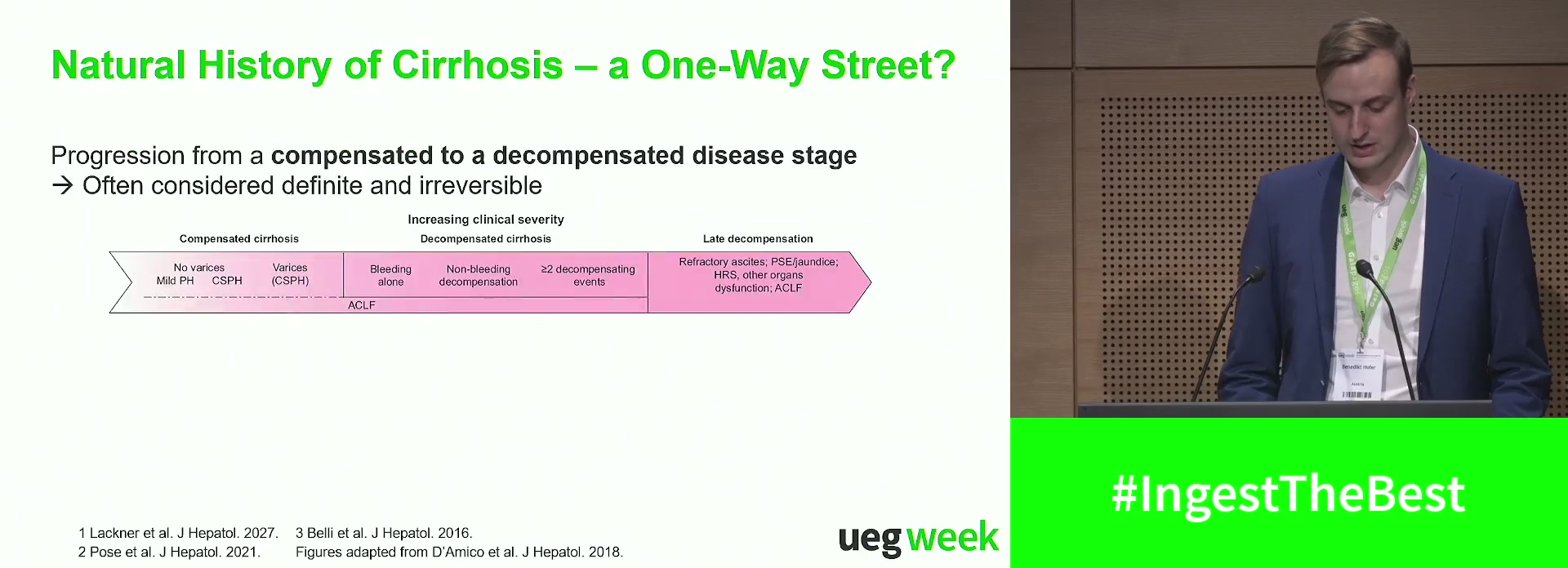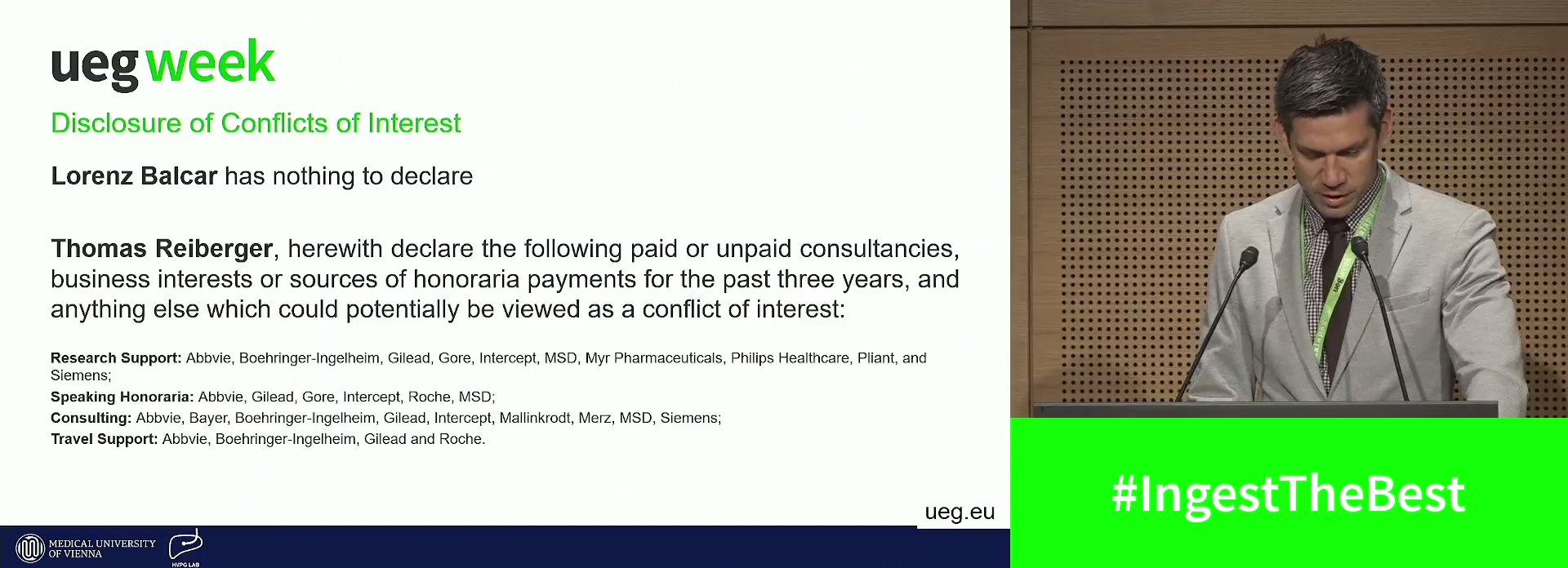### Mathias JachsMathias Jachs  et al.Mathias Jachs  et al.Mathias Jachs  et al.Mathias Jachs  et al.Mathias Jachs  et al.Mathias Jachs  et al.Mathias Jachs  et al.Mathias Jachs  et al.Mathias Jachs  et al.Mathias Jachs  et al.Mathias Jachs  et al.Mathias Jachs  et al.Mathias Jachs  et al.Mathias Jachs  et al.Mathias Jachs  et al.

Item 1 - 17 / 17